# The BriefA Blog about the LSAT, Law School and Beyond

Sometimes, the order of our objects does not matter. For example, suppose a professor is trying to assign people to different study groups. Then, it does not matter who gets assigned to the study group first; what matters is who is in which study groups, as in the following example:

Example
A professor is trying to split up his class of 9 students into 3 groups of 3. How many ways can he do this?

Or we might have:

Example
An appeals court is convening a 3-judge panel to hear a certain case. There are 26 judges in the courthouse. How many 3-judge panels are possible?

Now suppose that of the 26 judges, 13 are conservative and 13 are liberal. How many possible 3-judge panels will have at least one conservative and one liberal on the panel?

To address these kinds of problems, we will use the following:

Rule for Combinations
If you have n objects and you want to pick k of them to form a group, then there are n!/((n-k)!k!) ways to do so.

Let's try to solve our earlier example with this rule.

Solution
By applying our rule, we know that there areways of choosing 3 judges from the total group of 26 judges in order to form a panel. We can directly calculate this:, sinceand.
Thus,So there are 2600 ways to pick such a panel.

But why does this rule make sense? Well, let’s start with our rule for permutations: if I want to form a line of three judges from this group of 26, then there are 26*25*24 ways of doing this. This is because we have three spots to fill:

____  ____  ____

And for the first spot, we can pick any of the 26 judges; for the second, we can pick any of the remaining 25 judges, and for the last spot, we can pick any of the remaining 24 judges.

But here is the problem: we don’t actually care about the order in which we pick the judges. Suppose we have Judge Abby, Judge Ben, and Judge Cynthia. Then, we want to treat this possibility:

Abby Ben Cynthia

the same as this possibility:

Ben Abby Cynthia

which is in turn the same as

Cynthia Abby Ben

Cynthia Ben Abby

Abby Cynthia Ben

Ben Cynthia Abby.

We want to count all of these possibilities just once, since for us, a panel with Abby, Ben and Cynthia on it is just the same as a panel with Cynthia, Abby, and Ben on it. They’re not actually different!

Now, whenever we pick three judges from the 26 total judges, there are 3! ways to order those judges. This is because, for the first slot, we can pick any one of the three judges, for the second slot, we can pick any one of the remaining two judges, and for the last slot, we are stuck with the final judge.

So our permutation 26*25*24 actually counts each of these panels 3! times, when we only want to count each panel once. Suppose a panel has judge A, B, and C on it. Then our permutation counts:

ABC

ACB

BAC

BCA

CAB

CBA

So since our permutation is counting these panels 3! times when it should be counting them just once, we divide by 3! to get our final answer.

And, in general, the formula for how many combinations of k items we can pick from n objects is just equal to the number of permutations of k items we can pick from n objects, divided by (k!). Dividing by k! is how we make sure we are not counting all the different orders a particular combination can be put in.

Practice Problems

Question 1
A professor is trying to split up his class of 8 students into two groups of 4 students each. How many ways can he do this?

Question 2
Congress has decided to randomly pick a group of 4 congresspeople to lead a congressional committee. There are 535 congresspeople. How many groups are possible? What is the probability that any particular congressperson will be on the committee?

Question 3
Congress has decided to randomly pick a committee of 4 congresspeople, but they decide to pick as follows: 2 seats are randomly chosen from the Representatives while the remaining 2 are randomly chosen from the Senate. How many committees are possible? What is the probability that any particular Representative will be on the committee? What is the probability that any particular Senator will be on the committee?

Comment on this

And finally, we want to address the case when we have permutations with repeated objects. What if we have something like:

Example
The DMV is wondering how many new license plates it can form from just the letters: T, T, and R. How many such license plates are there?

Now, this question is something of a hybrid between our permutations and combinations. It is true that the order of the objects matters: TTR is not the same license plate as RTT. But we can’t just treat it as a standard permutation where we calculate n!/(n-k)!. If we tried that, we would get:

3!/(3-3)! = 3! = 6

But, if we list out all the possibilities, we find that we cannot form 6 different possibilities. The only possible license plates are:

TTR

TRT

RTT

You can try to think of another ordering, but there isn’t one. So treating it as a normal permutation leads us to the wrong answer. What is going on here?

To see what’s going wrong, let’s label our two T’s as T1 and T2. Now, let’s see how many ways we can order them:

T1 T2 R

T1 R T2

T2 T1 R

T2 R T1

R T1 T2

R T2 T1

Now we get the six possibilities we were expecting. But, remember, as a license plate, it doesn’t matter whether one uses T1 or T2. At the end of the day, the letter that shows up on the plate is just T. So, for example, combinations like R T1 T2 are just the same as R T2 T1. They both result in license plates that look like:

R T T

So, like in our combination problem, our permutation is over-counting things. It is treating as distinct some possibilities that are, in fact, the same. Here, our permutation gives us 6 possible outcomes. But, in fact, the ones with the same color are the same:

T1 T2 R

T1 R T2

T2 T1 R

T2 R T1

R T1 T2

R T2 T1

And we see that, in fact, we only have 3 different possibilities, which is what we found in listing out the possible license plates.

So our rule for these cases:

Permutations with Repeated Objects
Suppose you want to order k objects where one of the objects repeats n times. Then, the number of possible orderings is:.

is again a way of correcting for our over-counting. In our case, we can think of this rule as saying: there are 6 permutations. But within those permutations, the order of the T1 and T2 doesn’t matter. There are 2! ways to order T1 and T2, so the total number of distinct ways to order R, T, and T is just: 6/2! = 3.

Here is an application of this rule that involves larger numbers:

Example
You are playing Scrabble. Your hand has: T, R, S, S, S, E, and F. How many possible ways are there to order all of your tiles?

Solution
Following our rule, we want to find a way to order 7 objects (i.e. our 7 letters) where one of the objects is repeated 3 times. Then, there are 7!/3! ways to do this. And again,

7! = 7*6*5*4*3!

So,

7!/3! = 7*6*5*4 = 210

There are 210 possibilities

Practice Problems

Question 1
You are trying to come up with anagrams. You get the letters: A, Z, P, P, E, D. How many 6-letter combinations can be formed?

Question 2
You are playing Scrabble. You get the letters: S, S, S, S, T, E, P, X. How many 8-letter combinations can be formed?

Comment on this

So far we have talked about calculating probabilities of events where we tell you all the possible outcomes. For example, when we flip a coin, the possible outcomes are known: heads or tails. In such examples, you know how many possible outcomes there are. If we roll a six-sided die, there are clearly just six outcomes. But what if we are not given the number of possible outcomes? Consider this problem:

Example
There is a bag of 5 marbles; 3 blue and 2 red. Jane picks one marble out of the bag, looks at its color, sets it to the side (and does not return it to the bag) and picks another marble. What is the probability that she picks two blue marbles?

In this case, it isn’t clear how to apply our earlier rules. Remember our first rule:

Probability of Equally Likely Outcomes:
If you have n possible outcomes, all of which are equally likely, then the probability of any particular outcome occurring is 1/n.

How do we apply this rule to Jane’s situation? How many possible outcomes do we have?

This is where combinatorics comes in: it will allow us to determine how many possible outcomes we have. Now the word “combinatorics” is a little intimidating, but it’s just a fancy word for the mathematics of counting. In this case, we will use it to count the number of possible outcomes for Jane.

Let’s try to list out all the possible pairs of marbles that Jane could pick. To do this, let’s number our blue marbles 1 through 3, and number our red marbles 4 through 5. Now, Jane has to pick two different marbles. So, in other words, Jane ends up with some pair of marbles from the following list: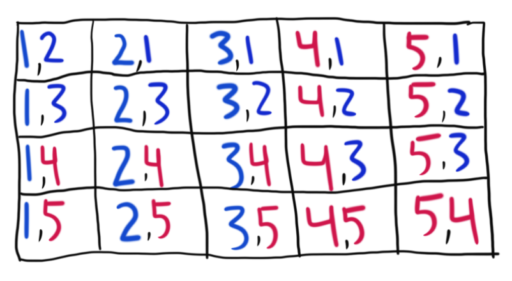So these are all the possible outcomes Jane could get. She could pick any of the 5 marbles first, and then she could pick any of the 4 remaining marbles second. Now, the question doesn’t say this explicitly, but we will assume (and it is generally fair game to assume) that Jane is equally likely to pick any of the marbles from the bag. After all, the marbles are presumably the same size and feel the same, so she’s just as likely to pick one as to pick any other.

Now, we have our set of equally likely outcomes. And these are all the outcomes. So, in line with our above rule, to find P(Jane picks 2 blue marbles) now we just need to pick out the outcomes where Jane picks two blue marbles. And that’s easy enough to see on our table, we just need to pick the outcomes where two blue marbles are chosen.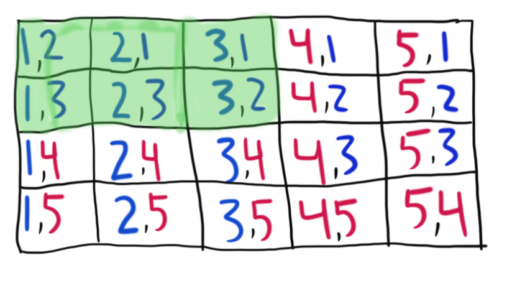Adding up these outcomes, we get 6/20 = 3/10. So that’s the likelihood of Jane picking two blue marbles.

In general, it will not be practical to make our table of possibilities and add things up. For if Jane had a bag of 10 marbles, we would have had 90 possible outcomes (10 choices for the first marble multiplied by 9 choices for the second). So, we introduce the following rule:

Rule for Permutations
Suppose we have n objects that we want to fill k spots where kn. Then, the number of possible ways ("permutations") to do this is:The n! is read as “n factorial” and it means n * (n-1) * (n-2) * … * (1). Basically, we take n and we multiply it by all the positive numbers less than or equal to it. Here are some examples:And, by a special convention, we say that 0! = 1.

Now, to solve probability questions, we want to find: how many permutations (or possible outcomes) will satisfy our event, and how many permutations in total are possible (i.e. how many possible outcomes there are). So in our above problem, we want to find:

1. How many permutations involve two blue marbles being drawn? (How many outcomes involve two blue marbles being drawn?)
We have three blue marbles, and we are drawing two marbles. So we want to use three objects to fill two spaces. Following our rule, we get:2. How many total permutations are there? (How many possible outcomes are there in total?)
We have five total marbles and we are drawing two of them. So we want to use five objects to fill two spaces. Following our rule, we get:Thus, we get: P(Draw two blue marbles) =, which matches our previous answer.

Practice Problems

Question 1
Suppose you have three friends: Anne, Beth, and Charles. How many ways can they form a three-person line?

Question 2
Mark is trying to descramble a series of letters: AQCFXDE. How many possible five-letter combinations can be formed from those letters?

Question 3
Jane is drawing four cards from a standard 52-card deck. What is the probability that she first draws the King of Hearts, and then the Ace of Spaces, and then the Jack of Clubs, and finally the Three of Hearts?

Comment on this

Initially, our Rule for Permutations may seem like an odd rule, but the following example may help to make it more intuitive. First recall:

Rule for Permutations
Suppose we have n objects that we want to fill k spots where kn. Then, the number of possible ways ("permutations") to do this is:Now consider:

Example
Our refrigerator has 5 magnetic numbers on it: 3, 2, 4, 5, and 7. How many ways are there to order the magnets on our fridge into 5-digit numbers?

Solution
By the question, we have 5 objects to fill into 5 spaces (corresponding to the five digits). Thus, by our formula, we get: 5!/(5-5)! = 5!/0! = 5!/1 = 5! = 120.

But to make this answer more intuitive, consider that we have five spaces to fill in with letters:

__               __               __               __               __

And for the first space, we can pick any number. It doesn’t matter which one. So we have five options for the first space.

Suppose we picked the number A. (A is just a stand-in for whatever number we actually picked; it doesn’t matter which one we picked). Then, we have:

(5 options)

And now, for the second digit, we can pick any number except for A. So we have four options. Suppose our second digit was B. Then we get:

(5 options)  (4 options)

And so on for the rest:

(5 options)  (4 options)   (3 options)   (2 options)  (1 option)

Thus, the total number of possibilities we could get is: 5 * 4 * 3 * 2 * 1 = 120, exactly what we got by following our rule.

Now, let’s see how permutations and probabilities can interact:

Example
You are forming four-digit numbers from the numbers 1, 2, 3, and 4. Each of your four-digit numbers must use all of those numbers exactly once. What is the probability that a number randomly chosen from your four-digit numbers contains a prime number in the tens place?

Solution
Now, we want to find:

How many four-digit numbers have a prime number in the tens place?

How many four-digit numbers are there?

To find (1), we want to consider numbers like:

(prime)

There are only two prime numbers here: 2, 3. Thus, all of our numbers must be like:

2

or

3

In either case, we have three choices for the remaining numbers (1, 3, 4 in the first case, and 1, 2, 4 in the second). Thus, there are 6 ways of ordering the remaining three in either case, so we have a total of 12 four-digit numbers with a prime in the tens place.

To answer (2), we want to find how many total four-digit numbers there are. This can be done via our rule: we just need to consider how many ways there are to put 4 objects into four slots. Thus, we getways to do so.

Thus, the answer is that there is achance of getting a prime in the tens place.

Comment on this

In a previous post, we discussed this rule:

Mutually Exclusive Rule for P(A or B)
Let A, B be mutually exclusive events. Then, P(A or B) = P(A) + P(B).

Here, we talk about why this rule makes sense. First, an example:

Example 1
In rolling a fair, six-sided die, what is the probability that you will get a 1 or a 4?

Solution
We know that rolling a 1 and rolling a 4 are mutually exclusive events, since it is impossible for them both to occur. We know that P(rolling a 1) = ⅙ and that P(rolling a 4) = ⅙. Thus, by our above rule, P(Rolling a 1 or rolling a 4) = ⅙ + ⅙ = ⅓.

We may also consider the different possible outcomes that are relevant here: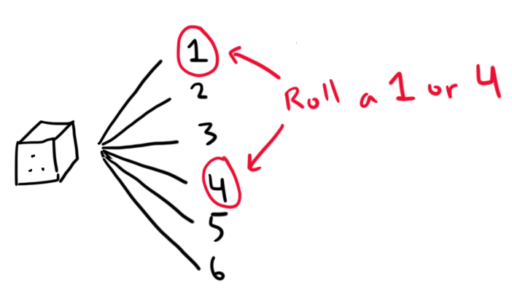So we roll a 1 or 4 in 2 of the six possible outcomes. Thus, the probability of doing so is just $\frac{2}{6} = \frac{1}{3}.$

In looking at some other examples, we may see why this more general rule makes sense:

General Rule for P(A or B)
Let A, B be two events. Then, P(A or B) = P(A) + P(B) - P(A and B).

Again, imagine we are rolling our favorite fair six-sided die. We are considering two events: A and B. We know that A occurs in three of the possible outcomes and B occurs in three of the possible outcomes. Can we determine P(A or B)? Now, this rule may seem a little arbitrary at first, but let’s see if we can see why it is true.

The answer is no. To see why, let’s consider two possible cases:

-       A: I roll an even number

-       B: I roll an odd number

Here, A occurs in three possible outcomes (2, 4, 6) and B occurs in three possible outcomes (1, 3, 5). And, in this case, P(A or B) = 1.

We can calculate this by considering how many of our outcomes satisfy A or B. So, for example, suppose we roll a 1. Then, “A or B” is true, since 1 is an odd number (so it is true that “I roll an odd number or I roll an even number”). Now suppose we roll a 2. Again, “A or B” is true, since 2 is an even number. Since all the numbers 1 through 6 are odd or even, we conclude that “A or B” will be true for all six outcomes. So, P(A or B) = 6/6 = 1.

But now consider this case:

-       C: I roll an even number

-       D: I roll a prime number

Again, C occurs in three possible outcomes (2, 4, 6) and D occurs in three possible outcomes (2, 3, 5). Now, in how many cases will “A or B” be true? Well let’s go through the cases. Suppose I roll a 1. Now, 1 is not even and it is not a prime number. So “C or D” will not be true if I roll a 1. Now consider 2; it is even, so “C or D” will be true if I roll a 2. And 3 is a prime number, 4 is even, 5 is prime, and 6 is even; so “C or D” will be true for all of those numbers.

Thus, P(A or B) = 5/6.

Thus, even when we know that A occurs in three of the possible outcomes and B occurs in three of the possible outcomes, we cannot determine P(A or B). (We are only able to do this if we get the additional information that A and B are mutually exclusive.)

To see why this is true, let’s picture each of the different outcomes as corresponding to some box.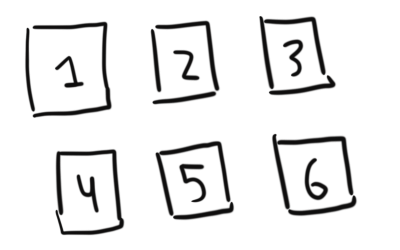Now, remember that for “A or B” to be true, we only need one of the two to be true. Let’s look at which boxes make A true.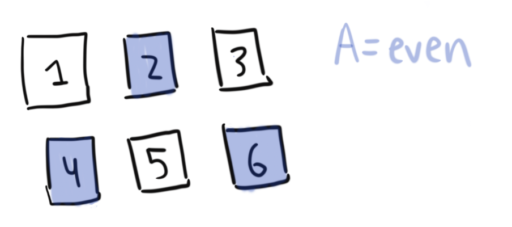And let’s now add which boxes make B true: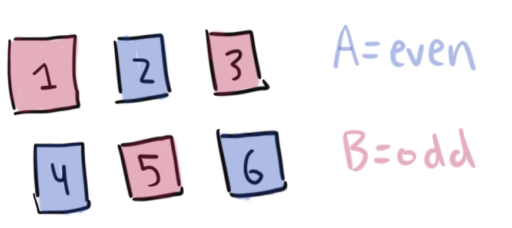So, the boxes that make “A or B” true will be all of the boxes.

But now let’s look at C and D. So, the boxes that make C true will be: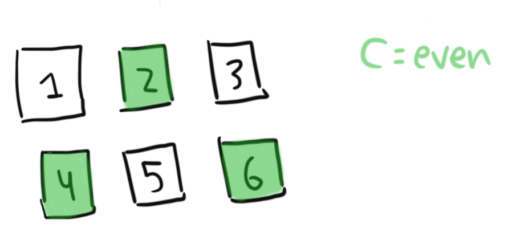And the boxes that make D true will be: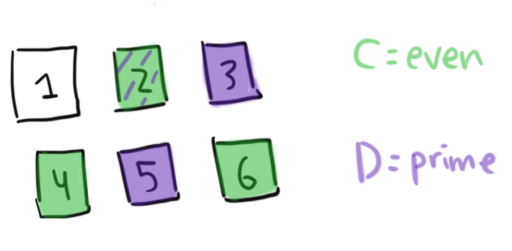Thus, the boxes that make “C or D” true will be only 5 of the 6 boxes. Looking at it this way, it becomes clear what went wrong: C and D overlap on one of the boxes. So we are “double-counting” the square 2. C counts it and D counts it. In some sense, we are wasting one of our boxes on 2.

That’s why our formula for calculating P(A or B) looks the way it does. First, we add the boxes where A is true. Second, we add the boxes where B is true.

But, now we’ve double-counted the boxes where A and B are both true – we added them once as part of A, and another time as part of B! That’s why our formula then has us subtract P(A and B). That way, we are cancelling out this double-counting.

So, to re-iterate, the formula for disjunctive probabilities is:

P(A or B) = P(A) + P(B) – P(A and B).

And from this general formula, we can derive the formula for Mutually Exclusive Disjunctions. So you only really need to learn this formula. How do we derive it?

Well, when A and B are mutually exclusive, we know that P(A and B) = 0. That’s just what it means to be mutually exclusive! Thus,

P(A or B) = P(A) + P(B) – P(A and B) = P(A) + P(B) – 0 = P(A) + P(B)

which was exactly our formula.

Comment on this

In a previous post, we discussed this formula:

Probabilities of Compound Events
Let A and B be independent of one another. Then, P(A and B) = P(A)P(B)

An example will help to illustrate why this formula makes sense.

Example
Suppose I roll a fair six-sided die and flip a fair coin. What is the probability that the coin lands heads and the die lands on six?

In general, listing out all the different possible outcomes will be infeasible. But it helps to get a sense for why this formula works. Here is an example where we wouldn’t bother to list out all the different outcomes:

Example
Suppose I roll three fair six-sided die in a row. What is the probability that the first die lands on an even number, and the second and third die both land on odd numbers?

Comment on this

Thus far, we have mostly talked about problems where all of the outcomes are equally likely. So, for example, our coins are equally likely to land heads as they are to land tails, and our spinners are equally likely to land on any of the outcomes, and our die are equally likely to land on any of 1 through 6. But not all GRE problems are like this. Consider the following:

Example 1
Suppose you have a weighted coin which is twice as likely to land tails as it is to land heads. You are about to flip this coin. What is the likelihood of it landing heads?

In these problems, we need to do a little bit of algebra to find the answer. Feel free to think about it, and if you want to see a solution, click below:

So in questions where the outcomes are unequal, we can use variables as placeholders for the true probabilities and then use the probability rules to derive the actual probabilities of the different outcomes. Here are some practice problems that are basically variations on this theme:

Practice Problems

Question 1
Suppose you have a weighted six-sided die that is four times as likely to land on the number 4 as it is to land on any of the other numbers. What is the probability that it lands on 6?

Question 2
Suppose you have a company raffle. Managers (of which there are 30) get 2 tickets, ordinary employees (of which there are 110) get 1 ticket, and executives (of which there are 5) get 20 tickets. What is the probability that the winner is an executive?

Question 3
Suppose you have an 8-sided spinner numbered 1 through 8. Now, your spinner is set up so that you are twice as likely to get 2 as you are to get 1, 3 times as likely to get 3 as your are to get 1, and so on for all the other numbers up until 8. What is the probability that you spin a 1?

Comment on this

Now, we discuss a trick that helps in some problems. Sometimes, instead of finding the probability of an event, it can be easier to find the probability that the event does not occur. Since we know that either an event will occur or not, we know that the probability of the event occurring plus the probability of the event not occurring must equal to 1. More formally, we write:

Probabilities for Complements
Let A be some event. Then, P(A) + P(A does not occur) = 1.

Here's an example where we apply this rule:

Example 1
You are about to roll a fair six-sided dice. What is the probability that you roll a prime number?

Why do we bother with this rule? Sometimes it is actually more convenient to calculate things this way. For example,

Example 2
You are going to roll a fair six-sided dice 10 times in a row. What is the probability that you get a six at least once?

Now, it will often be easier to just directly calculate the probability of a given event. But if that calculation looks absurdly difficult or tedious, take a minute to step back and consider: can I calculate the probability that the event doesn’t occur? Would that be easier? Sometimes that can save you a lot of hassle.

Practice Problems

Question 1
You are rolling a fair spinner with seven sections, numbered 1 through 7. What is the probability that, in 10 spins, you get at least one prime number?

Question 2
One random employee will be selected to win a company-sponsored vacation. There are 500 employees, 300 of which are female. Also, 50 employees are managers, and half of the managers are female. What is the probability that the winner is not a female manager?

Question 3
The weather reporters say that there is a 60% chance of rain for each of the next seven days. If that is true, what is the probability that it rains at some point over the next seven days?

Comment on this

Welcome to Law School Success Stories, where we discuss 7Sage applicants who made the most of their GPA and LSAT score.

👤 Who: “Sarah,” an applicant who grew up in China and moved to the United States for college.

• 📈 LSAT: 169
• 📉 GPA: 3.33

Results:

• 🏆 Accepted at the University of Michigan Law
• 💵 \$35,000 merit scholarship

🥅 Goals and Strategy

Sarah knew she wanted to take her law degree back to China, and the cachet of a T-14 school was important to her. Her parents, however, had a limited ability to pay for her education, and as a Chinese citizen, she wasn’t eligible for federal loans, so she was also hoping for a merit scholarship. Continue reading